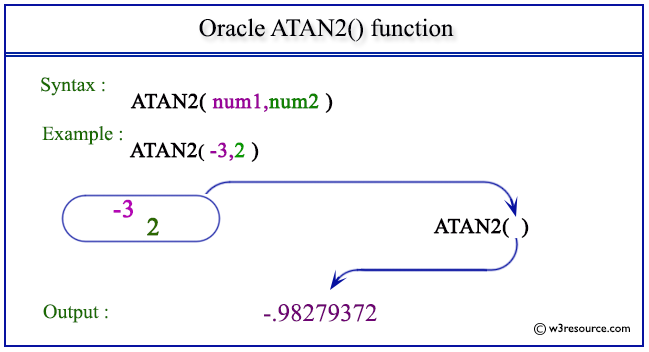# Oracle ATAN2() function

## Description

The ATAN2() function is used to calculate the arc tangent of two numbers for a point on a Cartesian plane. The first number in the argument can be in an unbounded range and returns a value in the range of -pi to pi, depending on the signs of num1 and num2 specified in the argument, expressed in radians.
The function takes any numeric or nonnumeric data type (can be implicitly converted to a numeric data type) as an argument.
If any argument is BINARY_FLOAT or BINARY_DOUBLE, then the function returns BINARY_DOUBLE. Otherwise, the function returns NUMBER.

Syntax:

`ATAN2(num1, num2);`

Arguments:

Name Description
num1 A number.
num2 A number.

Pictorial Presentation of ATAN2() functionExample1:

The statement below will return the arc tangent in radians of -3 and 2 for a point in a Cartesian plane.

``````SELECT ATAN2(-3,2) FROM dual;
```
```

Here is the result

```ATAN2(-3,2)
-----------
-.98279372
```

Example2

The statement below will return the arc tangent of .5 and .3 for a point in a Cartesian plane.

``````SELECT ATAN2(.5,.3) FROM dual;
```
```

Here is the result

```ATAN2(.5,.3)
------------
1.03037683
```

Previous: ATAN
Next: BITAND

﻿